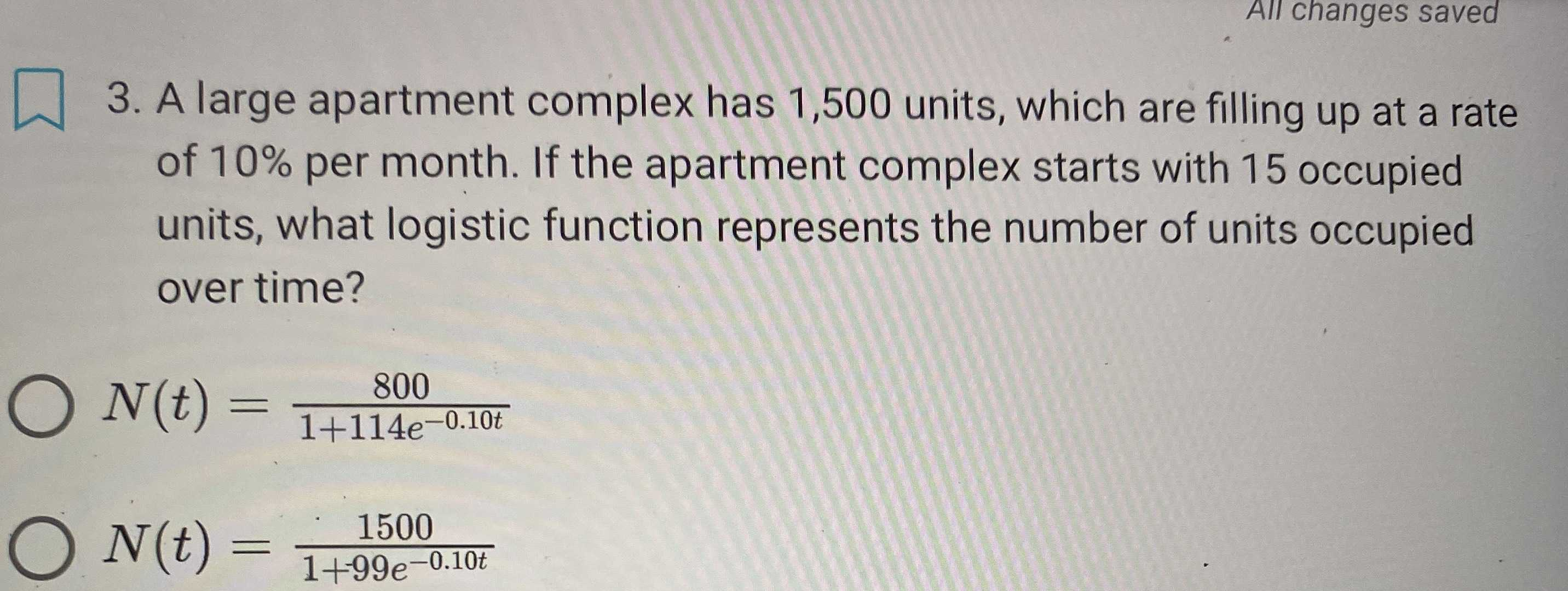### Still have math questions?

Algebra
QuestionA large apartment complex has $$1,500$$ units, which are filling up at a rate of $$10 \%$$ per month. If the apartment complex starts with $$15$$ occupied units, what logistic function represents the number of units occupied over time?

$$N ( t ) = \frac { 800 } { 1 + 114 e ^ { - 0.10 t } }$$

$$N ( t ) = \frac { 1500 } { 1 + 99 e ^ { - 0.10 t } }$$

$${ N ( t ) = \frac { 1500 } { 1 + 99 e ^ { - 0.10 t } } }$$